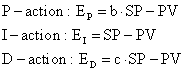﻿ 20-sim webhelp > Library > Signal > Control > PID Control > Initial Output

# Initial Output

The output of PID controllers at time 0 depends on the given setpoint (SP), process output (PV) and control law. In general the process will not be at rest (PV <> 0) and the setpoint will not be equal to the process output (SP <> 0). This will result in an undesired step in the controller signal at startup. Even if the setpoint is equal to the process output (SP = PV) at startup, the use of setpoint weighting may result in a step in the control signal:Let PV be a non zero value and b or c equal to zero. The P or D -action will then lead to a step in the control signal.

To avoid such a step, the initial output of some PID-controllers can be set to any value. The slope of the the control output of these controllers at startup is equal to zero.

## Initial output for with delays

Controllers with manual output at start-up, use the process output at t = 0, PV(0), to calculate an internal offset that compensates for the initial controller output. Care should be taken when delays are available between the process output and the controller input. Due to these, the measured process output at t = 0 in the controller ( PV(0)' ) may not be the same as the real process output at t = 0 ( PV(0) ). As a result, a wrong offset will be calculated and the initial output will not have the desired value. To avoid this, all initial values of delay elements between the process and controller should be chosen properly (e.g. equal to the initial process output) !